Worksheets and No Prep Teaching Resources
Math Worksheets

Learning addition math facts can be a chore, but not when combined with the creative activity of coloring sheets! These ingenious worksheets will help students easily learn basic math while creating a beautiful piece of art. Students will want to finish the engaging picture and will quickly master all addition facts that are so vital for future math success!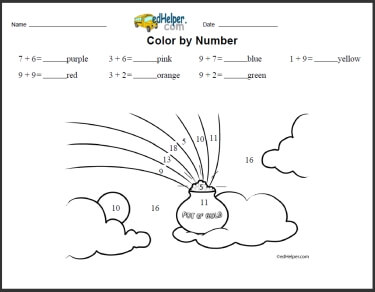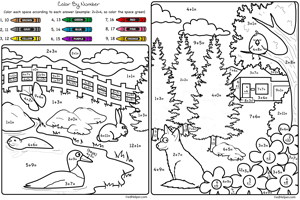Color by Number: Lake House

Math Lesson: Color by Numbers - February Color by Number Book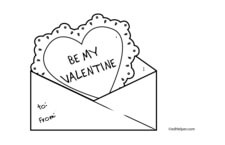Addition Coloring: an addition equation given for each color Addition and Subtraction Coloring: an addition or subtraction equation given for each color

Math Lesson: Color by Numbers - March Color by Number Book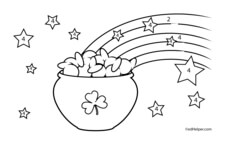Addition Coloring: an addition equation given for each color Addition and Subtraction Coloring: an addition or subtraction equation given for each color

Math Lesson: Color by Numbers - August Color by Number Book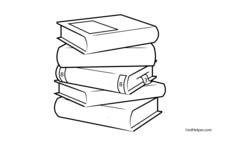Addition Coloring: an addition equation given for each color Addition and Subtraction Coloring: an addition or subtraction equation given for each color

Have a suggestion or would like to leave feedback?